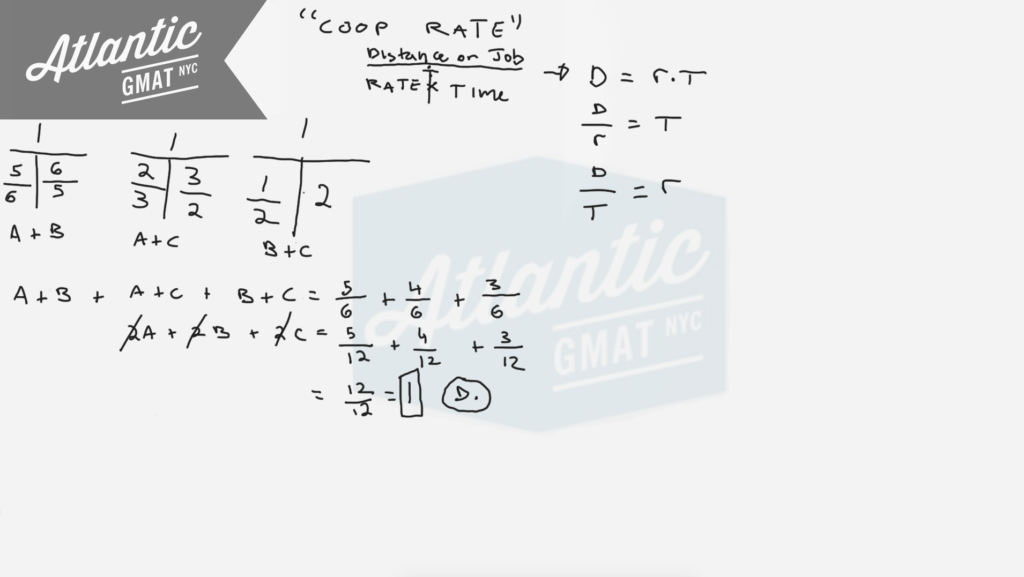Pumps A, B, and C operate at their respective constant rates. Pumps A and B, operating simultaneously, can fill a certain tank in 6/5 hours; pumps A and C, operating simultaneously, can fill the tank in 3/2 hours; and pumps B and C, operating simultaneously, can fill the tank in 2 hours. How many hours does it take pumps A, B, and C, operating simultaneously, to fill the tank.

(A) 1/3
(B) 1/2
(C) 1/4
(D) 1
(E) 5/6

This is a cooperative rate question so I would recommend using the T's to organize the information. It's a word problem so take your time reading and taking inventory of the question. Initially don't worry too much about the numbers. Pay attention to the structure and the question itself which you should define before doing any calculations. My first move is to splash out three T's to represent the three rates that were given, A + B, A + B, and B + C. We know that the rates need to be added because the pumps are operating simultaneously to fill the tank. What do we want to calculate: How many hours does it take pumps A, B, and C, operating simultaneously, to fill the tank? So we want to solve for the cooperative rate A + B + C  and then with that calculate the time it takes to get the tank filled. So, go ahead and add up the three sets of cooperative rates (5/6, 2/3, 1/2) and set them equal to the three cooperative rates they represent (A + B, A + C, B + C). Then group like terms and get common denominators for the fractions. You should end up with 2(A + B + C) = 2 or A + B + C = 1. The rate of 1 tank per hour means that the time it takes to fill the tank is, you guessed it: 1 hour.

Here are more Official GMAT distance, work, and rate question for which we use the T method:

Circular gears P and Q start rotating at the same time at constant speeds

A boat traveled upstream a distance of 90 miles at an average speed of (v-3) miles per hour and then traveled the same distance downstreamHere are another bunch of cooperative work and rate questions to practice on:

Work and Rate 1

Work and Rate 2

Work and Rate 3

Work and Rate 4

Work and Rate 5

For more distance, work, and rate examples you can also check out our GMAT Question of the Day page

# CONTACT

Atlantic GMAT

405 East 51st St.

NY, NY 10022

(347) 669-3545

info@AtlanticGMAT.com